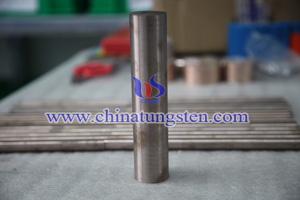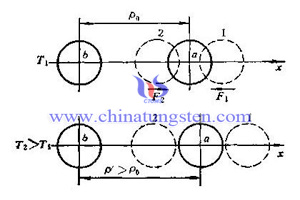Tungsten Copper Rod>>
Tungsten Copper>>
What is Tungsten Copper>>
Tungsten Copper Properties>>
Tungsten Copper Application>>
Tungsten Copper Machining>>
Tungsten Copper Products>>
Tungsten Copper Electrode>>
Tungsten Copper Heat Sink>>
Tungsten Copper Contacts>>
Tungsten Copper FGM>>
Tungsten Copper AlN>>
Other Copper Alloy Products>>
Customer Service and Support>>

# Tungsten Copper Rod CTE

## Introduction

Tungsten copper rod CTE (Coefficient of Thermal Expansion) represents the variation of length by temperature changes under constant pressure. And the unit of metal CTE is 1/℃.

## Type

Tungsten copper rod CTE can be divided into linear expansion coefficient α, superficial expansivity β and volume expansion coefficient γ.
When the temperature change is not very large, α becomes a constant, it can represent both solid and liquid volume expansion as follow: Vt = V0 (1 + 3αΔT), Vt = V0 (1 + 0.00367ΔT) for ideal gas; Vt and V0 represent the volume of end state and initial state individually.
For the object can be regarded as one-dimensional, the length is the determinant to measure the volume, and CTE can be defined as: the ratio of the increasing length and original length at certain temperature, which is called as linear expansion coefficient.
For three-dimensional anisotropic substance, it can be divided into linear expansion coefficient and volume expansion coefficient. Graphite fiber linear displays expansion coefficient anisotropicity, expressed as a direction parallel to the level of thermal expansion coefficient much smaller than the dimension perpendicular to the direction.

## Function

1. Improve the thermal stability of tungsten copper alloy;
2. Improve the strength of tungsten copper alloy;
3. It is beneficial for welding or dissolving;## Measurement

With the increasing temperature, the thermal expansion of tungsten copper passed to displacement sensor by mandrel in the furnace. And displacement amount measured by sensor is the thermal expansion displacement. The system will change the temperature signal and the displacement signal transmitted in real-time data acquisition and processing by PC, respectively, to calculate the thermal expansion coefficient of the material obtained by the thermal expansion of the equation, which is the basic principle of thermal expansion measurements.